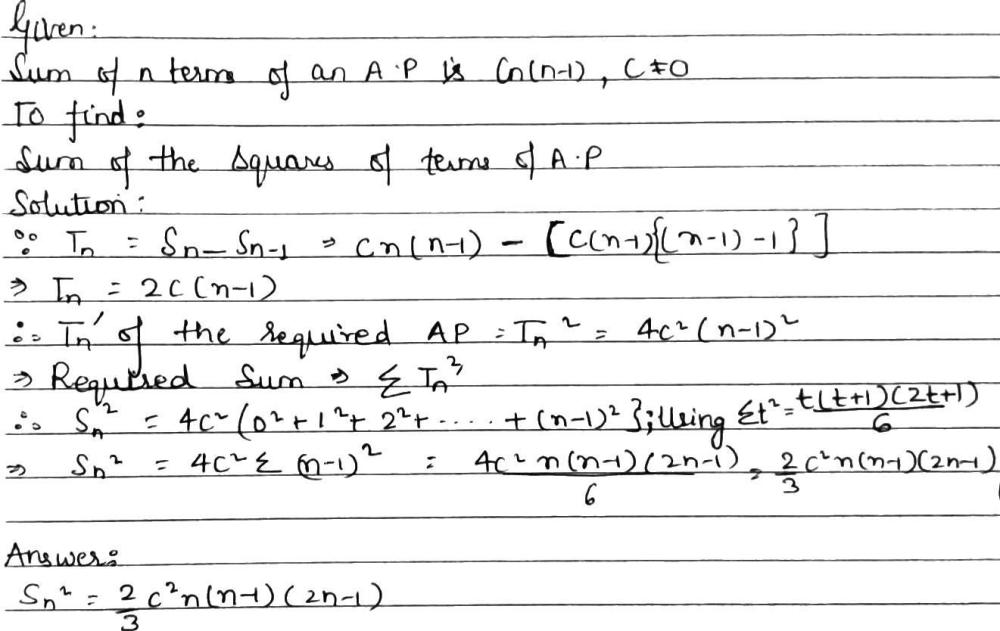Home/Class 11/Maths/

## QuestionMathsClass 11

If the sum of n terms of an A.P. is cn$$\left(n - 1\right)$$ where c $$\ne\;0$$, then the sum of the squares of these terms is
A.$${c}^{2}n{\left(n + 1\right)}^{2}$$
B.$$\frac{2}{3}{c}^{2}n\left(n - 1\right)\left(2n - 1\right)$$
C.$$\frac{{2c}^{2}}{3}n\left(n+ 1\right)\left(2n+ 1\right)$$
D.none of these